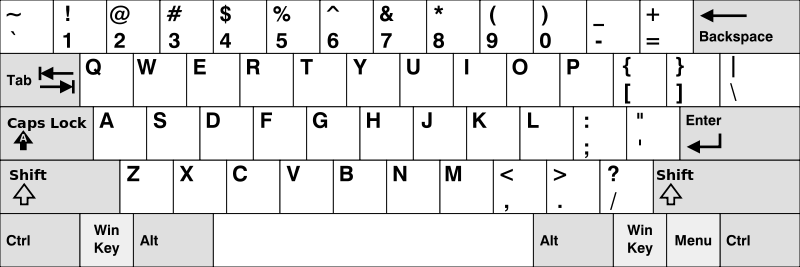﻿ 【算法千题案例】每日LeetCode打卡——79.键盘行

### 【算法千题案例】每日LeetCode打卡——79.键盘行

2021-11-18 4:27:09

• 📢前言
• 🌲原题样例：键盘行
• 🌻C#方法：排序遍历
• 🌻Java 方法：计数
• 💬总结#### 📢前言

 🚀 算法题 🚀
• 🌲 每天打卡一道算法题，既是一个学习过程，又是一个分享的过程😜
• 🌲 提示：本专栏解题 编程语言一律使用 C# 和 Java 两种进行解题
• 🌲 要保持一个每天都在学习的状态，让我们一起努力成为算法大神吧🧐！
• 🌲 今天是力扣算法题持续打卡第79天🎈！
 🚀 算法题 🚀

## 🌲原题样例：键盘行

• 第一行由字符 “qwertyuiop” 组成。
• 第二行由字符 “asdfghjkl” 组成。
• 第三行由字符 “zxcvbnm” 组成。``````输入：words = ["Hello","Alaska","Dad","Peace"]

``````

``````输入：words = ["omk"]

``````

``````输入：words = ["adsdf","sfd"]

``````

• 1 <= words.length <= 20
• 1 <= words[i].length <= 100
• words[i] 由英文字母（小写和大写字母）组成

### 🌻C#方法：排序遍历

• 我们可以预处理计算出每个字符对应的行号。

• 遍历字符串时，统一将大写字母转化为小写字母方便计算。

``````public class Solution {
public string[] FindWords(string[] words) {
IList<string> list = new List<string>();
string rowIdx = "12210111011122000010020202";
foreach (string word in words) {
bool isValid = true;
char idx = rowIdx[char.ToLower(word) - 'a'];
for (int i = 1; i < word.Length; ++i) {
if (rowIdx[char.ToLower(word[i]) - 'a'] != idx) {
isValid = false;
break;
}
}
if (isValid) {
}
}

string[] ans = new string[list.Count];
for (int i = 0; i < list.Count; ++i) {
ans[i] = list[i];
}
return ans;
}
}
``````

``````通过

``````

### 🌻Java 方法：计数

• 我们可以预处理计算出每个字符对应的行号。

• 遍历字符串时，统一将大写字母转化为小写字母方便计算。

``````class Solution {
public String[] findWords(String[] words) {
List<String> list = new ArrayList<String>();
String rowIdx = "12210111011122000010020202";
for (String word : words) {
boolean isValid = true;
char idx = rowIdx.charAt(Character.toLowerCase(word.charAt(0)) - 'a');
for (int i = 1; i < word.length(); ++i) {
if (rowIdx.charAt(Character.toLowerCase(word.charAt(i)) - 'a') != idx) {
isValid = false;
break;
}
}
if (isValid) {
}
}
String[] ans = new String[list.size()];
for (int i = 0; i < list.size(); ++i) {
ans[i] = list.get(i);
}
return ans;
}
}
``````

``````通过

``````

## 💬总结

• 今天是力扣算法题打卡的第七十九天！
• 文章采用 `C#``Java` 两种编程语言进行解题
• 一些方法也是参考力扣大神写的，也是边学习边分享，再次感谢算法大佬们
• 那今天的算法题分享到此结束啦，明天再见！